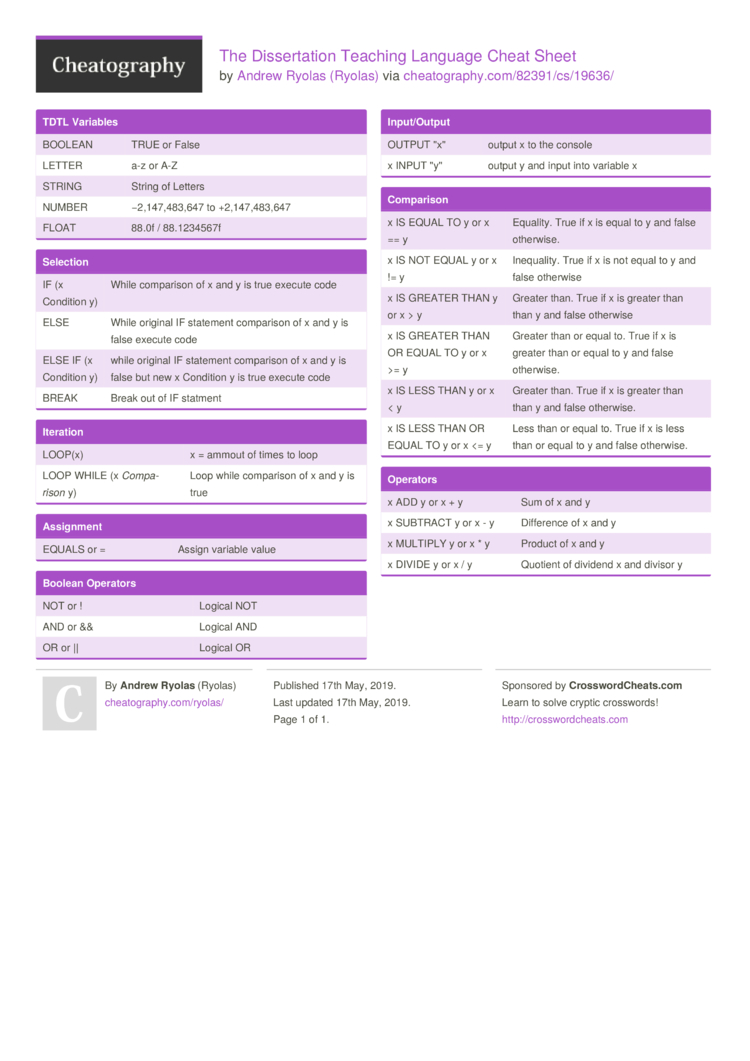# The Dissertation Teaching Language Cheat Sheet by Ryolas

### TDTL Variables

 BOOLEAN TRUE or False LETTER a-z or A-Z STRING String of Letters NUMBER −2,147­,48­3,647 to +2,147­,48­3,647 FLOAT 88.0f / 88.123­4567f

### Selection

 IF (x Condition y) While comparison of x and y is true execute code ELSE While original IF statement comparison of x and y is false execute code ELSE IF (x Condition y) while original IF statement comparison of x and y is false but new x Condition y is true execute code BREAK Break out of IF statment

### Iteration

 LOOP(x) x = ammout of times to loop LOOP WHILE (x Compa­rison y) Loop while comparison of x and y is true

### Assignment

 EQUALS or = Assign variable value

### Boolean Operators

 NOT or ! Logical NOT AND or && Logical AND OR or || Logical OR

### Input/­Output

 OUTPUT "­x" output x to the console x INPUT "­y" output y and input into variable x

### Comparison

 x IS EQUAL TO y or x == y Equality. True if x is equal to y and false otherwise. x IS NOT EQUAL y or x != y Inequa­lity. True if x is not equal to y and false otherwise x IS GREATER THAN y or x > y Greater than. True if x is greater than than y and false otherwise x IS GREATER THAN OR EQUAL TO y or x >= y Greater than or equal to. True if x is greater than or equal to y and false otherwise. x IS LESS THAN y or x < y Greater than. True if x is greater than than y and false otherwise. x IS LESS THAN OR EQUAL TO y or x <= y Less than or equal to. True if x is less than or equal to y and false otherwise.

### Operators

 x ADD y or x + y Sum of x and y x SUBTRACT y or x - y Difference of x and y x MULTIPLY y or x * y Product of x and y x DIVIDE y or x / y Quotient of dividend x and divisor y1 Page
//media.cheatography.com/storage/thumb/ryolas_the-dissertation-teaching-language.750.jpg

PDF (recommended)